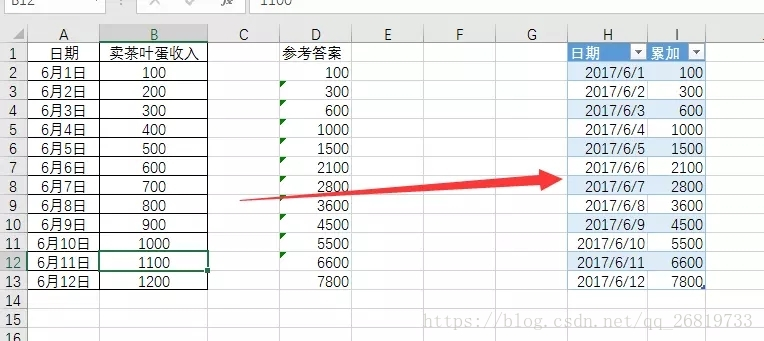# Excel / SQL实现按日期累加``````select a.日期,
(
select sum(b.卖茶叶蛋收入) as 累加 from
[sheet1\$a1:b13] b where b.日期<=a.日期
)  as 累加
from
[sheet1\$a1:b13] a
``````

``````select a.日期,sum(b.卖茶叶蛋收入) as 累加 from
[sheet1\$a1:b13] a
inner join
[sheet1\$a1:b13] b
on
a.日期>=b.日期
group by a.日期``````